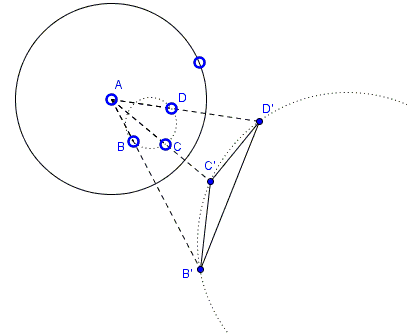# Ptolemy's Equality/Inequlity via Inversion

### Ptolemy's Theorem

For four distinct coplanar points $A,$ $B,$ $C,$ and $D,$

1. $AB\cdot CD+AD\cdot BC\ge AC\cdot BD.$

2. The equality only reached when the four points are concyclic.

### Hint

There are several known proofs of this well known and useful result; the applet above was intended to suggest that inversion might help furnish another proof.

### Proof

A proof is available on a separate page. Below are just two illustrations:### Ptolemy's Theorem